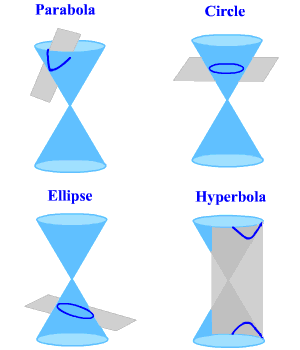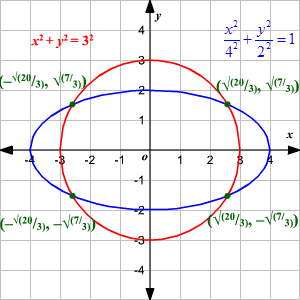# Conic Sections and Standard Forms of Equations

A conic section is the intersection of a plane and a double right circular cone .  By changing the angle and location of the intersection, we can produce different types of conics. There are four basic types: circles , ellipses , hyperbolas and parabolas .    None of the intersections will pass through the vertices of the cone.If the right circular cone is cut by a plane perpendicular to the axis of the cone, the intersection is a circle.  If the plane intersects one of the pieces of the cone and its axis but is not perpendicular to the axis, the intersection will be an ellipse.  To generate a parabola, the intersecting plane must be parallel to one side of the cone and it should intersect one piece of the double cone. And finally, to generate a hyperbola the plane intersects both pieces of the cone. For this, the slope of the intersecting plane should be greater than that of the cone.

The general equation for any conic section is

$A{x}^{2}+Bxy+C{y}^{2}+Dx+Ey+F=0$ where $A,B,C,D,E$ and $F$ are constants.

As we change the values of some of the constants, the shape of the corresponding conic will also change.  It is important to know the differences in the equations to help quickly identify the type of conic that is represented by a given equation.
If ${B}^{2}-4AC$ is less than zero, if a conic exists, it will be either a circle or an ellipse.
If ${B}^{2}-4AC$ equals zero, if a conic exists, it will be a parabola.
If ${B}^{2}-4AC$ is greater than zero, if a conic exists, it will be a hyperbola.

## STANDARD FORMS OF EQUATIONS OF CONIC SECTIONS:

 Circle ${\left(x-h\right)}^{2}+{\left(y-k\right)}^{2}={r}^{2}$ Center is $\left(h,k\right)$ . Radius is $r$ . Ellipse with horizontal major axis $\frac{{\left(x-h\right)}^{2}}{{a}^{2}}+\frac{{\left(y-k\right)}^{2}}{{b}^{2}}=1$ Center is $\left(h,k\right)$ . Length of major axis is $2a$ . Length of minor axis is $2b$ . Distance between center and either focus is $c$ with ${c}^{2}={a}^{2}-{b}^{2},a>b>0$ . Ellipse with vertical major axis $\frac{{\left(x-h\right)}^{2}}{{b}^{2}}+\frac{{\left(y-k\right)}^{2}}{{a}^{2}}=1$ Center is $\left(h,k\right)$ . Length of major axis is $2a$ . Length of minor axis is $2b$ . Distance between center and either focus is $c$ with ${c}^{2}={a}^{2}-{b}^{2},a>b>0$ . Hyperbola with horizontal transverse axis $\frac{{\left(x-h\right)}^{2}}{{a}^{2}}-\frac{{\left(y-k\right)}^{2}}{{b}^{2}}=1$ Center is $\left(h,k\right)$ . Distance between the vertices is $2a$ . Distance between the foci is $2c$ . ${c}^{2}={a}^{2}+{b}^{2}$ Hyperbola with vertical transverse axis $\frac{{\left(y-k\right)}^{2}}{{a}^{2}}-\frac{{\left(x-h\right)}^{2}}{{b}^{2}}=1$ Center is $\left(h,k\right)$ . Distance between the vertices is $2a$ . Distance between the foci is $2c$ . ${c}^{2}={a}^{2}+{b}^{2}$ Parabola with horizontal axis ${\left(y-k\right)}^{2}=4p\left(x-h\right)$ , $p\ne 0$ Vertex is $\left(h,k\right)$ . Focus is $\left(h+p,k\right)$ . Directrix is the line $x=h-p$ Axis is the line $y=k$ Parabola with vertical axis ${\left(x-h\right)}^{2}=4p\left(y-k\right)$ , $p\ne 0$ Vertex is $\left(h,k\right)$ . Focus is $\left(h,k+p\right)$ . Directrix is the line $y=k-p$ . Axis is the line $x=h$

## Solving Systems of Equations

You must be familiar with solving system of linear equation . Geometrically it gives the point(s) of intersection of two or more straight lines. In a similar way, the solutions of system of quadratic equations would give the points of intersection of two or more conics.

Algebraically a system of quadratic equations can be solved by elimination or substitution just as in the case of linear systems.

Example:

Solve the system of equations.

$\begin{array}{l}{x}^{2}+4{y}^{2}=16\\ {x}^{2}+{y}^{2}=9\end{array}$

The coefficient of ${x}^{2}$ is the same for both the equations. So, subtract the second equation from the first to eliminate the variable $x$ . You get:

$3{y}^{2}=7$

Solving for $y$ :

$\begin{array}{l}\frac{3{y}^{2}}{3}=\frac{7}{3}\\ \text{\hspace{0.17em}}\text{\hspace{0.17em}}\text{\hspace{0.17em}}{y}^{2}=\frac{7}{3}\\ \text{\hspace{0.17em}}\text{\hspace{0.17em}}\text{\hspace{0.17em}}\text{\hspace{0.17em}}\text{\hspace{0.17em}}y=±\sqrt{\frac{7}{3}}\end{array}$

Use the value of $y$ to evaluate $x$ .

$\begin{array}{l}{x}^{2}+\frac{7}{3}=9\\ {x}^{2}=9-\frac{7}{3}\\ \text{\hspace{0.17em}}\text{\hspace{0.17em}}\text{\hspace{0.17em}}\text{\hspace{0.17em}}\text{\hspace{0.17em}}\text{\hspace{0.17em}}=\frac{20}{3}\\ \text{\hspace{0.17em}}\text{\hspace{0.17em}}x=±\sqrt{\frac{20}{3}}\end{array}$

Therefore, the solutions are $\left(+\sqrt{\frac{20}{3}},+\sqrt{\frac{7}{3}}\right),\left(+\sqrt{\frac{20}{3}},-\sqrt{\frac{7}{3}}\right),\left(-\sqrt{\frac{20}{3}},+\sqrt{\frac{7}{3}}\right)$ and $\left(-\sqrt{\frac{20}{3}},-\sqrt{\frac{7}{3}}\right)$ .

Now, let us look at it from a geometric point of view.

If you divide both sides of the first equation ${x}^{2}+4{y}^{2}=16$ by 16 you get $\frac{{x}^{2}}{16}+\frac{{y}^{2}}{4}=1$ . That is, it is an ellipse centered at origin with major axis $4$ and minor axis $2$ . The second equation is a circle centered at origin and has a radius $3$ . The circle and the ellipse meet at four different points as shown.